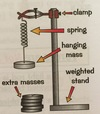Materials Flashcards Preview

Physics > Materials > Flashcards

Flashcards in Materials Deck (28):
1

2

3

4

5

6

7

what does Hooke's law look like graphically?

A is the elastic limit so the line is no longer straight afterwards8

9

10

over which range does the spring obey Hooke's law? summarise the characteristsics of the graph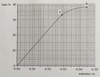11

sketch a graph showing a single spring, a two springs in series and a two parallel springs being extended

A = single spring being extendedB = series spring being extendedC = parallel spring being extended12

if a single spring (A) being extended has a gradient of 0.250 N/cm then calculate the gradient of two springs in series (B) and two parallel springs (C) being extended

A (single) = 0.250 N/cmB (series) = 0.125 N/cmC (parallel) = 0.500 N/cm13

14

describe the relationship of Agradient of A = 0.250 N/cm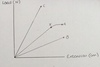15

describe the relationship of Bcompare it to to Agradient of A = 0.250 N/cmgradient of B = 0.125 N/cm16

describe the relationship of Ccompare it to to Agradient of A = 0.250 N/cmgradient of C = 0.500 N/cm17

rank the springs in order of strength (strongest first, weakest last)18

19

D is an elastic banddescribe the elastic band by referring to the graph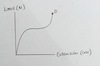20

draw a clear diagram of the equipment used in an experiment  investing Hooke's law on a single spring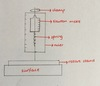21

select equipment for investigating Hooke's law on a single spring

The experiment is set up as shown. A retort stand is attached to a clamp holding a Newton metre which is holding a spring. Another clamp holds a ruler. The spring is pulled down (extended). Each time the spring is extended one centimetre further. The load is recorded after each extension using the Newton metre. control variable = the surface the experiment is carried out onindependent variable = the extension of the spring, measured by a ruler in cmdependent variable = the load on the spring, measured by a Newton metre in Newtons (N)22

23

24

25

26

27

28

How do you investigate Hooke's law with a spring?

1. Set up the apparatus. Make sure you have plenty of extra masses and measure the weight of each (with a balance)2. Measure the length of the spring (e.g. with an accurate mm ruler) when no load is applied. Ensure the ruler is vertical (e.g. set square) and measure the spring at eye level. (This is the spring's natural level)3. Add one mass at a time and allow the spring to come to rest, then measure the new length of the spring. The extension is the change in the length from the original length. Adding a marker to the top and bottom of the spring might make measuring lengths easier. Repeat this process until you have enough measurements (no fewer than 6)4. Once you're done, repeat the experiment and calculate an average value for the length of the spring for each applied weight. This will make your results reliable